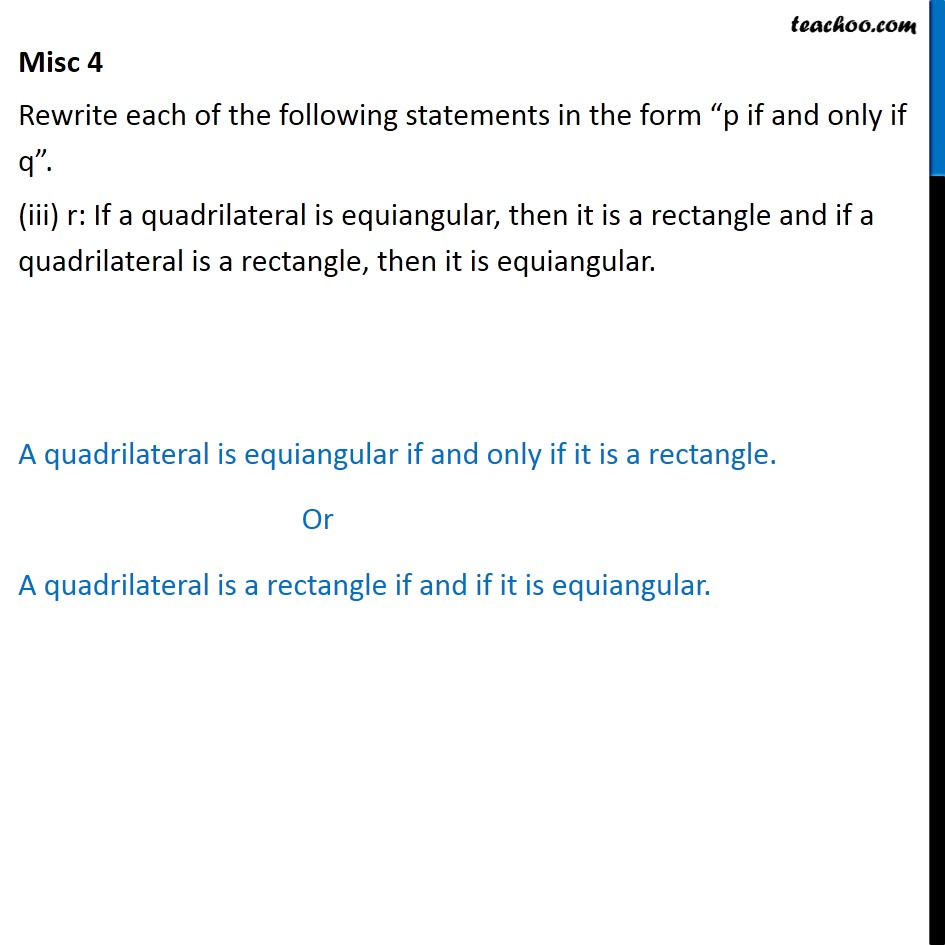Miscellaneous

Mathematical Reasoning
Serial order wiseLearn in your speed, with individual attention - Teachoo Maths 1-on-1 Class

### Transcript

Misc 4 Rewrite each of the following statements in the form “p if and only if q”. (iii) r: If a quadrilateral is equiangular, then it is a rectangle and if a quadrilateral is a rectangle, then it is equiangular. A quadrilateral is equiangular if and only if it is a rectangle. Or A quadrilateral is a rectangle if and if it is equiangular.